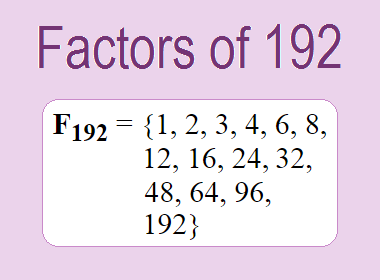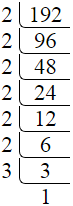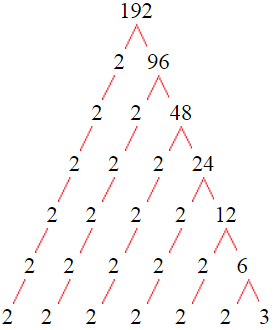# Factors of 192The factors of 192 are 1, 2, 3, 4, 6, 8, 12, 16, 24, 32, 48, 64, 96, and 192 i.e. F192 = {1, 2, 3, 4, 6, 8, 12, 16, 24, 32, 48, 64, 96, 192}. The factors of 192 are all the numbers that can divide 192 without leaving a remainder.

We can check if these numbers are factors of 192 by dividing 192 by each of them. If the result is a whole number, then the number is a factor of 192. Let's do this for each of the numbers listed above:

·        1 is a factor of 192 because 192 divided by 1 is 192.

·        2 is a factor of 192 because 192 divided by 2 is 96.

·        3 is a factor of 192 because 192 divided by 3 is 64.

·        4 is a factor of 192 because 192 divided by 4 is 48.

·        6 is a factor of 192 because 192 divided by 6 is 32.

·        8 is a factor of 192 because 192 divided by 8 is 24.

·        12 is a factor of 192 because 192 divided by 12 is 16.

·        16 is a factor of 192 because 192 divided by 16 is 12.

·        24 is a factor of 192 because 192 divided by 24 is 8.

·        32 is a factor of 192 because 192 divided by 32 is 6.

·        48 is a factor of 192 because 192 divided by 48 is 4.

·        64 is a factor of 192 because 192 divided by 64 is 3.

·        96 is a factor of 192 because 192 divided by 96 is 2.

·        192 is a factor of 192 because 192 divided by 192 is 1.

## How to Find Factors of 192?

1 and the number itself are the factors of every number. So, 1 and 192 are two factors of 192. To find the other factors of 192, we can start by dividing 192 by the numbers between 1 and 192. If we divide 192 by 2, we get a remainder of 0. Therefore, 2 is a factor of 192. If we divide 192 by 3, we get a remainder of 0. Therefore, 3 is also a factor of 192.

Next, we can check if 4 is a factor of 192. If we divide 192 by 4, we get a remainder of 0. Therefore, 4 is also a factor of 192. If we divide 192 by 5, we get a remainder of 2. Therefore, 5 is not a factor of 192. We can continue this process for all the possible factors of 192.

Through this process, we can find that the factors of 192 are 1, 2, 3, 4, 6, 8, 12, 16, 24, 32, 48, 64, 96, and 192. These are the only numbers that can divide 192 without leaving a remainder.

## Properties of the Factors of 192

The factors of 192 have some interesting properties. One of the properties is that the sum of the factors of 192 is equal to 508. We can see this by adding all the factors of 192 together:

1 + 2 + 3 + 4 + 6 + 8 + 12 + 16 + 24 + 32 + 48 + 64 + 96 + 192 = 508

Another property of the factors of 192 is that the prime factors of 192 are 2, and 3 only.

## Applications of the Factors of 192

The factors of 192 have several applications in mathematics. One of the applications is in finding the highest common factor (HCF) of two or more numbers. The HCF is the largest factor that two or more numbers have in common. For example, to find the HCF of 192 and 120, we need to find the factors of both numbers and identify the largest factor they have in common. The factors of 192 are 1, 2, 3, 4, 6, 8, 12, 16, 24, 32, 48, 64, 96, and 192. The factors of 120 are 1, 2, 3, 5, 6, 8, 10, 12, 15, 20, 24, 30, 40, 60, and 120. The largest factor that they have in common is 24. Therefore, the HCF of 192 and 120 is 24.

Another application of the factors of 192 is in prime factorization. Prime factorization is the process of expressing a number as the product of its prime factors. The prime factors of 192 are 2, and 3 since these are the only prime numbers that can divide 192 without leaving a remainder. Therefore, we can express 192 as:

192 = 2 × 2 × 2 × 2 × 2 × 2 × 3

We can do prime factorization by division and factor tree method also. Here is the prime factorization of 192 by division method,192 = 2 × 2 × 2 × 2 × 2 × 2 × 3

Here is the prime factorization of 192 by the factor tree method,192 = 2 × 2 × 2 × 2 × 2 × 2 × 3

## Conclusion

The factors of 192 are the numbers that can divide 192 without leaving a remainder. The factors of 192 are 1, 2, 3, 4, 6, 8, 12, 16, 24, 32, 48, 64, 96, and 192. The factors of 192 have some interesting properties, such as having a sum of 508. The factors of 192 have several applications in mathematics, such as finding the highest common factor and prime factorization.